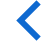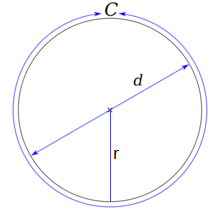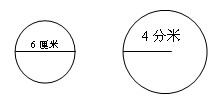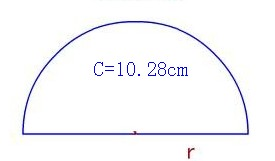##圆的周长公式

C=πd=2πr

C：表示圆的周长;d：表示圆的直径;r：表示圆的半径。如下图：1：解：已知圆的直径为6厘米，即：

d=6cm;

C=πd=3.14 x 6=18.84(cm)

2：解：已知圆的半径为4分米，即：

r=6dm;

C=2πr=2x 3.14 x 4=25.12(dm)2r+πr=10.28

r(2+π)=10.28

r=10.28÷(2+π)

r=2(cm)

C=2πr=2 x 3.14 x 2=12.56cm

• 球体的体积公式
• 球体的表面积公式
• 圆的面积公式
• 圆的周长公式